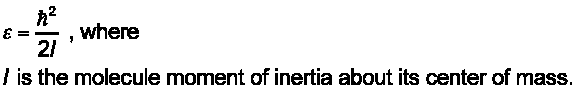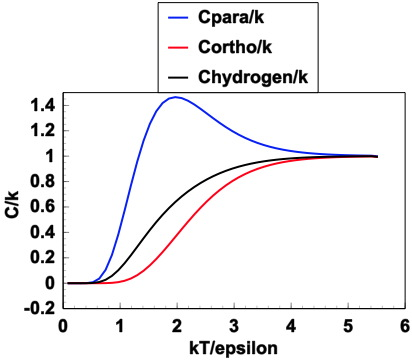PHYSICS 425 & 625 Homework [example] [fortran on the mac] [return to main page]

 Extra Credit: Problem 6.21. Heat capacity of the anharmonic hydrogen molecule, and interpretation of results. You can modify the code we used for problem 6.26 and apply it here for this problem. Make a plot similar to the one we did for that problem. However, do an overlay of 1. the harmonic oscillator solution when En=Epsilon*n , 2. the heat capacity when you sum to n=10 in the partition function, 3. the heat capacity when you sum to n=12 in the partition function, 4. the heat capacity when you sum to n=15 in the partition function.

 Homework 17 Problems 7.19 and 7.43. Properties of Fermions and Bosons, i.e. electrons in a metal and photons from the sun.

 Homework 16 Read chapter 7, carefully, with concentration, effort, and strength. Answer the following questions. 1. How is the Gibbs factor different from the Boltzmann factor? 2. Why is it that quantum statistics are different for particles called Bosons and Fermions? 3. What keeps matter from collapsing under the huge electrostatic forces that try to pull electrons and protons together? 4. What is the ultraviolet catastrophe? 5. How does the total energy density of a black body vary with temperature? Think about the Earth at a lowly 285 K, the Sun exterior at 5800 K, and the Sun interior at around 15 million K. 6. How does the entropy of a black body relate to the number of photons in a black body? 7. What is the value of the Stefan Boltzmann constant? Use it to estimate how much radiative power your body would emit (neglecting clothes). How many food calories would you need to eat to replace the energy you would lose in 12 hours due to black body radiation emission if you did not receive infrared energy from the environment. 8. How is the Debye theory of solids different from the Einstein theory of solids? 9. What is Bose Einstein condensation?

 Homework 15 Problems 6.33, 6.48 . Speed of O2 molecules at room temperature. Then the partition function for O2, and obtain the entropy and chemical potential from it. Problem 6.24 helps with the rotational partition function for O2 at room temperature.

 Homework 14 (due date moved). Problem 6.26. Rotational partition function at low temperature. Note thatOR Problem 6.30, parts (a), (b), and (c). Computational problem concerning the heat capacity of para and ortho hydrogen. You will have to figure out how to take the derivative for heat capacity calculation from energy. You can use Mathematica or Maple and do the derivatives using these programs, or do so numerically using other programs such as Fortran or Matlab, etc. Fortran example. Matlab example. Perl language example. Another Matlab example. (someone send me a C-code or other example too.) Your results should look something like this:OR You can do both with one for extra credit.

 Homework 13 Problems 6.3 and 6.10. In 6.3 it is a good idea to plot the partition function as a function of (kT/epsilon) too so you can see the functional form. Boltzmann's constant is given in eV/K on page 402. On problem 6.10, you can calculate the partition function by adding terms, and can quit adding terms when they are so small that they don't contribute anymore. On problem 6.10, calculate the wavelength of a photon (in micron units) that corresponds to an excitation from the ground state to the first excited state; what part of the electromagnetic spectrum is this photon in?

 Homework 12 Read Chapter 6 very very thoroughly. Answer the following questions. 1. How is the Boltzmann factor defined. Why is it useful? 2. What does the partition function partition? 3. What assumption(s) must be made when deriving the equipartition theorem? 4. Sketch the Maxwell speed distribution. Why don't all molecules in an ideal gas just have the same average speed? 5. What is the relationship between the partition function and the Helmholtz free energy?

 Homework 11 Problem 3.34. Statistical and thermal properties of rubber bands. SOLUTION.

 Homework 10 Problem 3.7. Black hole temperature. Hint: Should find a very small T. Problem 3.10. Note that the 'universe' here is the kitchen and the ice and water. Part d is the entropy sum of parts a through c. SOLUTION.

 Homework 9 Read chapter 3. Answer the following conceptual questions: 1. What is temperature (refined answer from our chapter 1 definition)? 2. How do you calculate entropy from heat capacity? 3. What is the 'third law' and how does it affect entropy? 4. Do you agree with the author's silly economic/entropy analogy? 5. What are negative absolute temperatures? 6. What are the conditions for mechanical equilibrium in two systems? 7. What is diffusive equilibrium? 8. What is the chemical potential? 9. What are the conditions of diffusive equilibrium? 10. Write down equations for obtaining temperature, pressure, and chemical potential from partial derivatives of entropy.

 Homework 8 Entropy of mixing, entropy of a black hole. Black hole: truth and lies. Black hole: just two numbers is all you need... Black holes: Tall, Grande, Vente. (more on black holes.) Black hole tutorial. Problems 2.37, 2.42

 Homework 7 Entropy of an ideal gas: derivation and application. Problems 2.31, 2.33

 Homework 6 Stirling approximation for large multiplicities, and physics of a random walk. Problems 2.18, 2.25 parts a and b only. On part b, it may be helpful to look at the daily notes.

 Homework 5 Statistics of a two state system (like a spin system in a magnetic field) and an 'Einstein' solid. Problems 2.3, 2.7

 Homework 4 Read chapter 2. Pay particular attention to the concepts. Turn in these questions for review: 1. What is a microstate? 2. What is a macrostate? 3. Write down the fundamental assumption of Statistical Mechanics. Do you agree with it? Explain. 4. What is the author's physical explanation of heat? Do you agree? 5. The author gives a statement of the second law of thermodynamics. Write it down. Do you agree with it? 6. What is the Stirling approximation? Why is it useful? 7. What is the thermodynamic limit? 8. Write down the fundamental equation for entropy and define all the terms. Discuss this definition. What do large values of entropy imply? Small values? 9. Give an example of a reversible and irreversible process. 10. Read problems 2.41, 2.42, and 2.43 carefully, and think about them.

 Homework 3 Latent heat and heat transfer. (Begin reading chapter 2 when finished). Problems 1.48, 1.61, and 1.62. Problem 1.62 is mandatory for grad students signed up for Phys 625; it is optional as extra credit for undergrads in Phys 425. Below is a discussion of problem 1.62. On problem 1.61 it is useful to look at the peak solar radiation absorbed at mid day to get a feel for the comparison with heat transfer rate in the Earth. Recent neutrino detection experiments are now being used to study theEarth's internal heating mechanisms (radioactive decay) (here is the fascinating article.)   Mathematica code for the plot: Plot[Evaluate[ Table[Exp[-x^2/(4 t)]/Sqrt[t], {t, .25, 5, 0.25}]], {x, -10, 10}, PlotRange -> {{-10, 10}, {0, 2}}, AxesLabel -> {"x", "T(x)" - SubscriptBox[T, 0] // DisplayForm}, Frame -> False, BaseStyle -> {FontWeight -> "Bold", FontSize -> 14}] Code for the animation: Animate[Plot[Exp[-x^2/(4 t)]/Sqrt[t], {x, -10, 10}, Filling -> Axis, FillingStyle -> Red, PlotRange -> {{-10, 10}, {0, 2}}, AxesLabel -> {"x", "T(x)" - SubscriptBox[T, 0] // DisplayForm}, Frame -> False, BaseStyle -> {FontWeight -> "Bold", FontSize -> 14}], {t, 0.25, 5, 0.1}] Try with Mathematica Online.

 Homework 2 due Thursday 15 September Student solution for HW2. Heat Capacity, Enthalpy, Work, Heat, cyclic processes, atmospheric thermodynamics. READING ASSIGNMENT: Read chapter 1. Devour sections 1.4 through 1.6 for this first assignment. After doing the assignment, read chapter 2. QUESTIONS FOR REVIEW (copy and paste these to your word processor, answer them, and turn them in with the problems): a. Why is enthalpy so useful? b. What is the enthalpy of formation of H20, starting with H2 and 1/2O2 in the gaseous form? Endothermic or exothermic reaction? c. Why if I put 1 Joule of energy into various systems they all don't have the same temperature change? Do problems: 1.34 (Illustrates cyclic processes like used for heat engine analysis) 1.37 (Thoughts about how diesel engines work) 1.40 (Calculate the dry adiabatic lapse rate for the atmosphere) 1.43 (Think about the degrees of freedom available to a liquid. Why?)

 Homework 1 due Tuesday 6 September Temperature. Here is a student solution for HW1. The purpose of this assignment is to think about temperature, perhaps in new ways. READING ASSIGNMENT: Read chapter 1. Devour sections 1.1 through 1.3 for this first assignment. Bring questions from the reading to class!!! QUESTIONS FOR REVIEW (copy and paste these to your word processor, answer them, and turn them in with the problems): a. What is temperature? Give three definitions. b. What is meant by thermal equilibrium? Can we do thermodynamics on systems that are not in thermal equilibrium? c. What are two mechanisms for objects to use to come to thermal equilibrium? d. In your experience, what is the relaxation time necessary for a hot cup of coffee, tea, or water to come to thermal equilibrium with at room at room temperature? e. What is the most exotic type of thermometer you can think of? f. What would you rather touch, a piece of fluffy carpet or a large piece of aluminum, if both are at 353 K? Why? PROBLEMS: Do problems 1.7, 1.8. On problem 1.7 you do not have to get a mercury thermometer. Just make an estimate of its size and design one to your liking. Comment on why might we not want to use mercury for a thermometer. Also, extra credit for a thorough discussion of why mercury is a liquid rather than solid at typical room conditions, in comparison with its nearest neighbors in the periodic table.

Problems for homework and class discussion: 1.32, 1.34, 1.37, 1.40, 1.42, 1.43, 1.46, 1.47, 1.48, 1.50, 1.51, 1.54.
You can start thinking about them now.